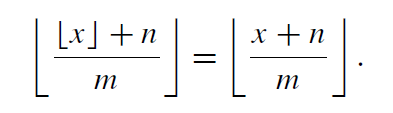×
Get Full Access to Discrete Mathematics And Its Applications - 7 Edition - Chapter 2.se - Problem 26e
Get Full Access to Discrete Mathematics And Its Applications - 7 Edition - Chapter 2.se - Problem 26e

×

Prove that if m and n are positive integers and x is aISBN: 9780073383095 37

Solution for problem 26E Chapter 2.SE

Discrete Mathematics and Its Applications | 7th Edition

• Textbook Solutions
• 2901 Step-by-step solutions solved by professors and subject experts
• Get 24/7 help from StudySoup virtual teaching assistantsDiscrete Mathematics and Its Applications | 7th Edition

4 5 1 417 Reviews
25
0
Problem 26E

Prove that ifandare positive integers andis a real number, thenStep-by-Step Solution:
Step 1 of 3

Solution:

Step1

Given that

We have to prove that if m and n are positive integers and x is a real number, thenStep2

We have m and n are positive integers and x is a real number.

Suppose x= b+y where b is an integer andThen we have

L.H.S(left hand side)

R.H.S(right hand side)

HereStep3Put value of x= b+y we getPut value of x= b+y we getAsTherefore, L.H.S=R.H.SStep 2 of 3

Step 3 of 3

ISBN: 9780073383095

Unlock Textbook Solution